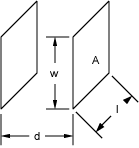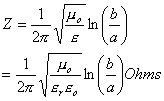NESS ENGINEERING
TECHNICAL DATA
IMPEDANCE

The formulas on this page allow one to calculate the impedance for certain given geometries.  More complex problems typically require one to model the geometry and solve using Finite Element Analysis.

2 Parallel Plane Platesm0 is the permeability (H/m) e is the permittivity (F/m)  e0 is the permittivity of free space (F/m)  er is the relative permittivity of the dielectric  d is the distance between the electrodes (m) w is the width of the electrodes (m)

2 Coaxial Cylindersm0 is the permeability (H/m) e is the permittivity (F/m)  e0 is the permittivity of free space (F/m)  er is the relative permittivity of the dielectric   a is the radius of the inner cylinder  b is the radius of the outer cylinder

Send consulting inquiries, comments, and suggestions to nessengr@san.rr.com

Ness Engineering, Inc.
P.O. Box 261501
San Diego, CA 92196
(858) 566-2372
(858) 240-2299 FAX

 © Richard M. Ness and Ness Engineering, Inc. Last Updated: